# RD Sharma Solutions for Class 6 Maths Chapter 11: Angles Exercise 11.1

In our day to day life, we come across many physical objects having two arms joined together by a hinge. Some of the examples are two arms of a divider, two sharp parts of scissors, two hands of a clock etc. These objects give us an idea about an angle in geometry, as discussed in this Chapter. An angle is a figure formed by two rays with the same initial point. In order to know more about the concepts which are covered in this Chapter, the students can refer to RD Sharma Solutions Class 6 Maths Chapter 11 Angles Exercise 11.1 PDF which are provided here.

## RD Sharma Solutions for Class 6 Maths Chapter 11: Angles Exercise 11.1 Download PDF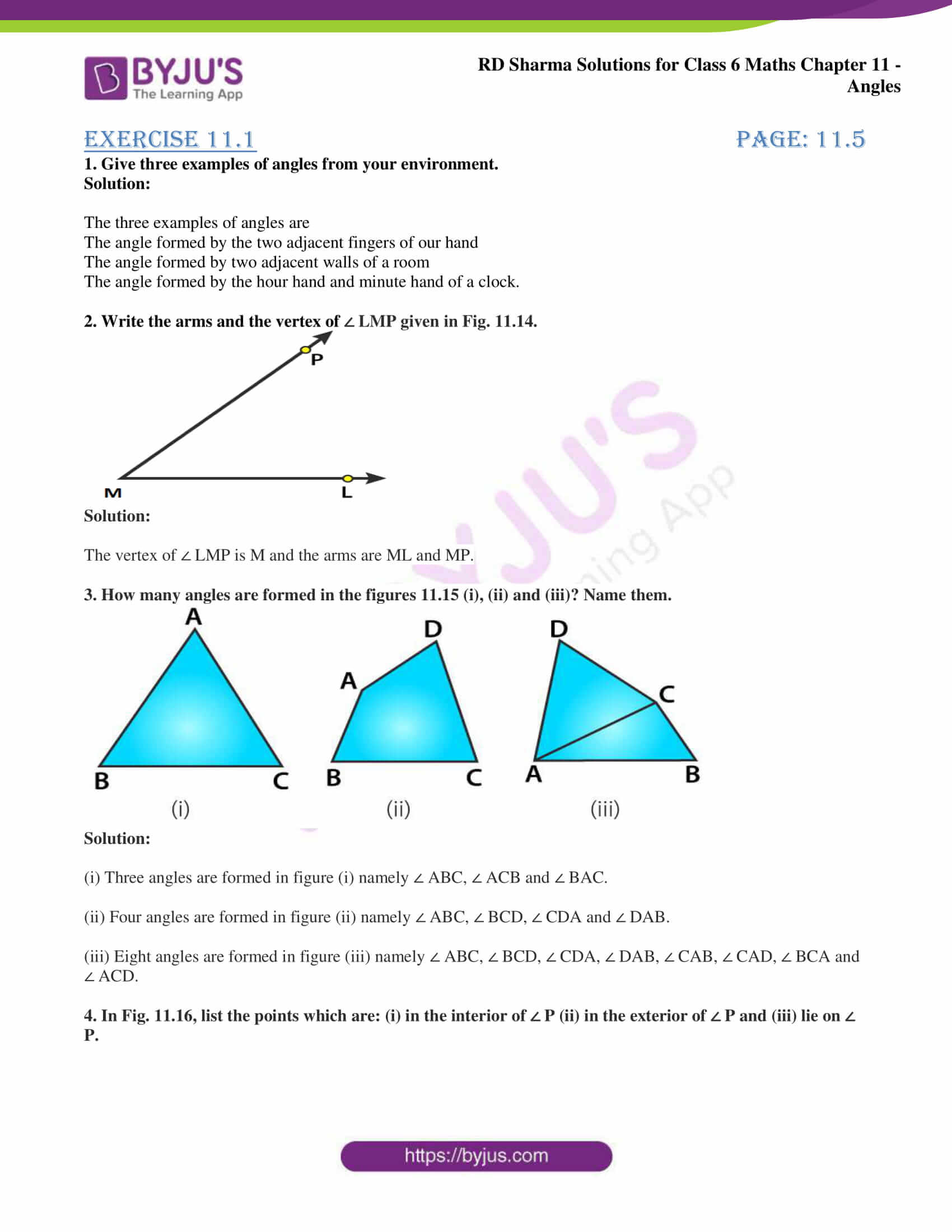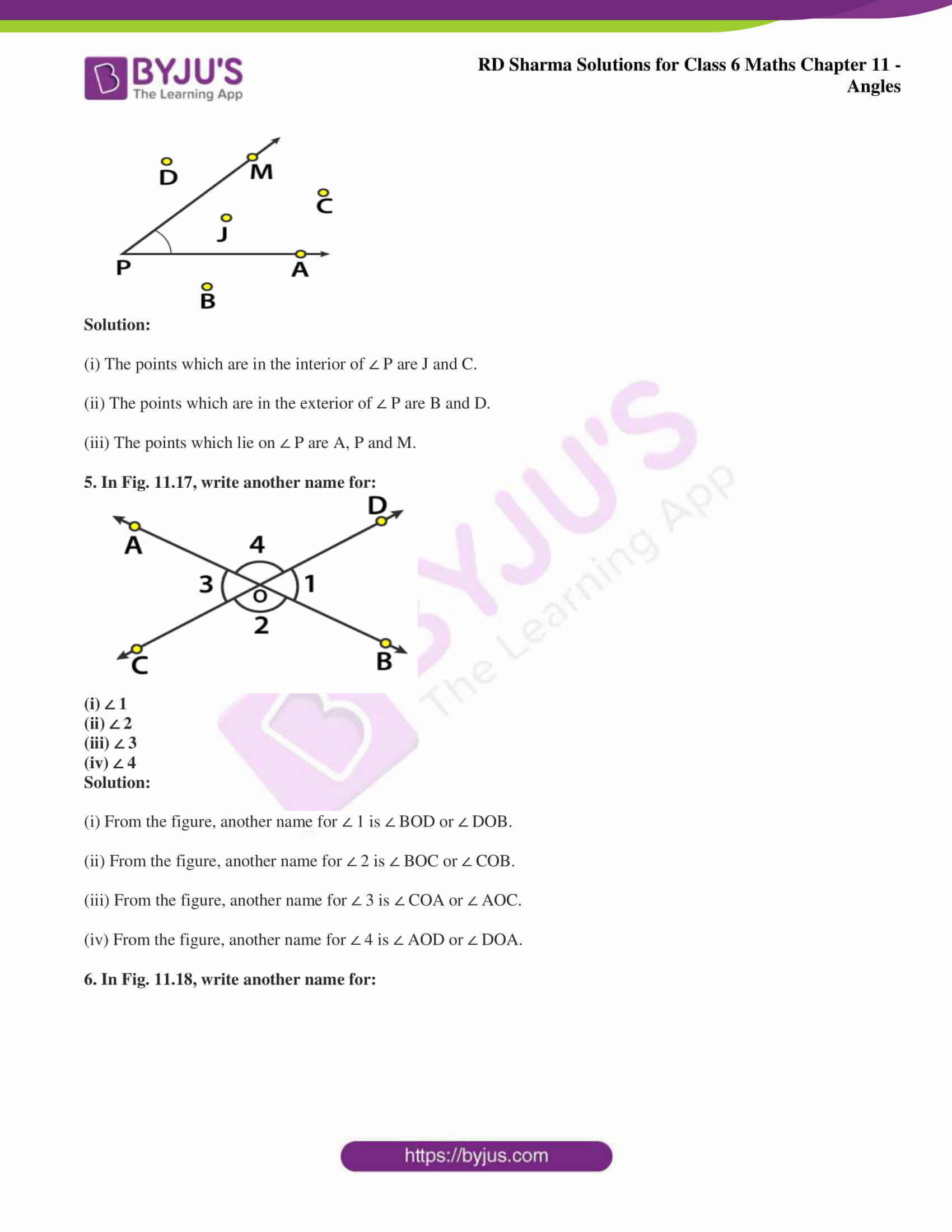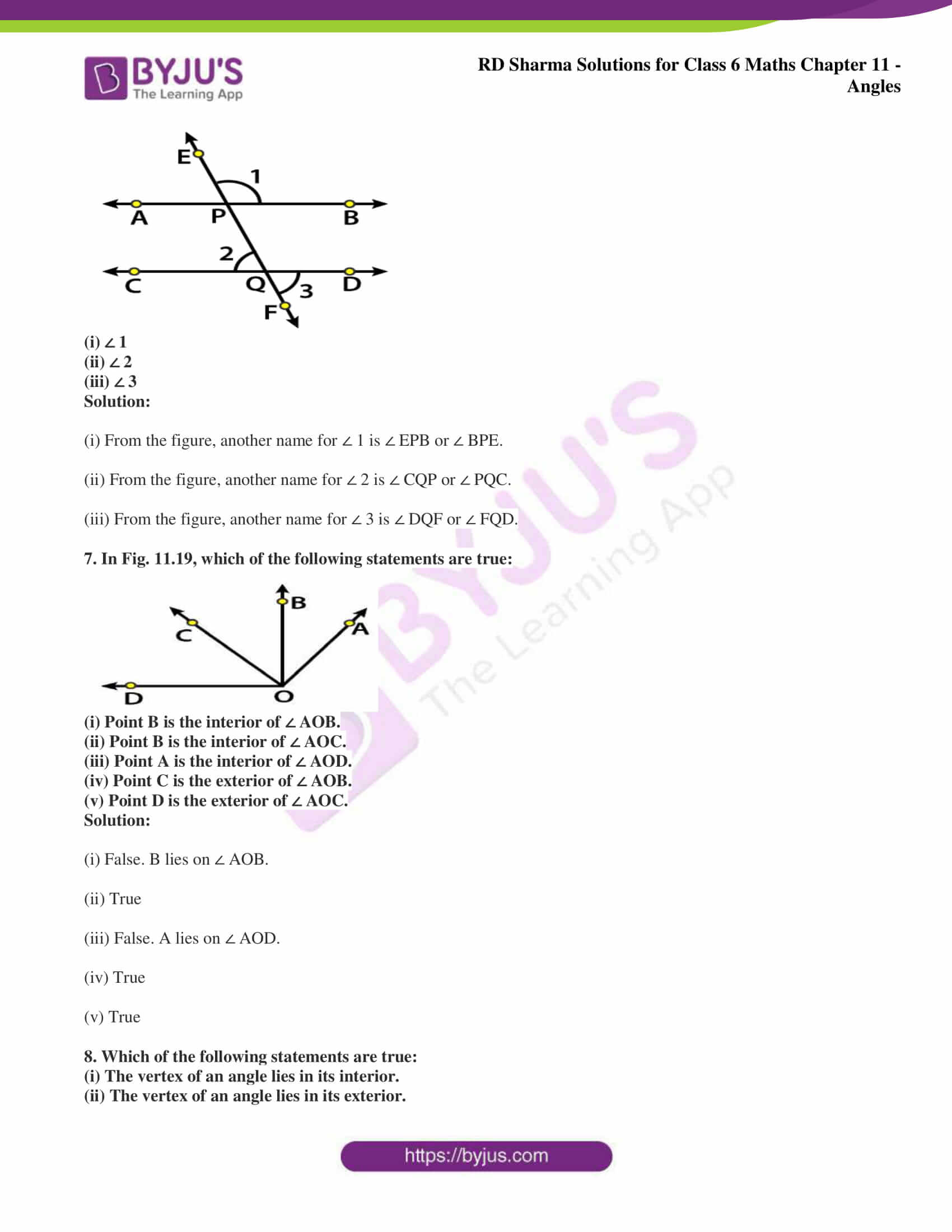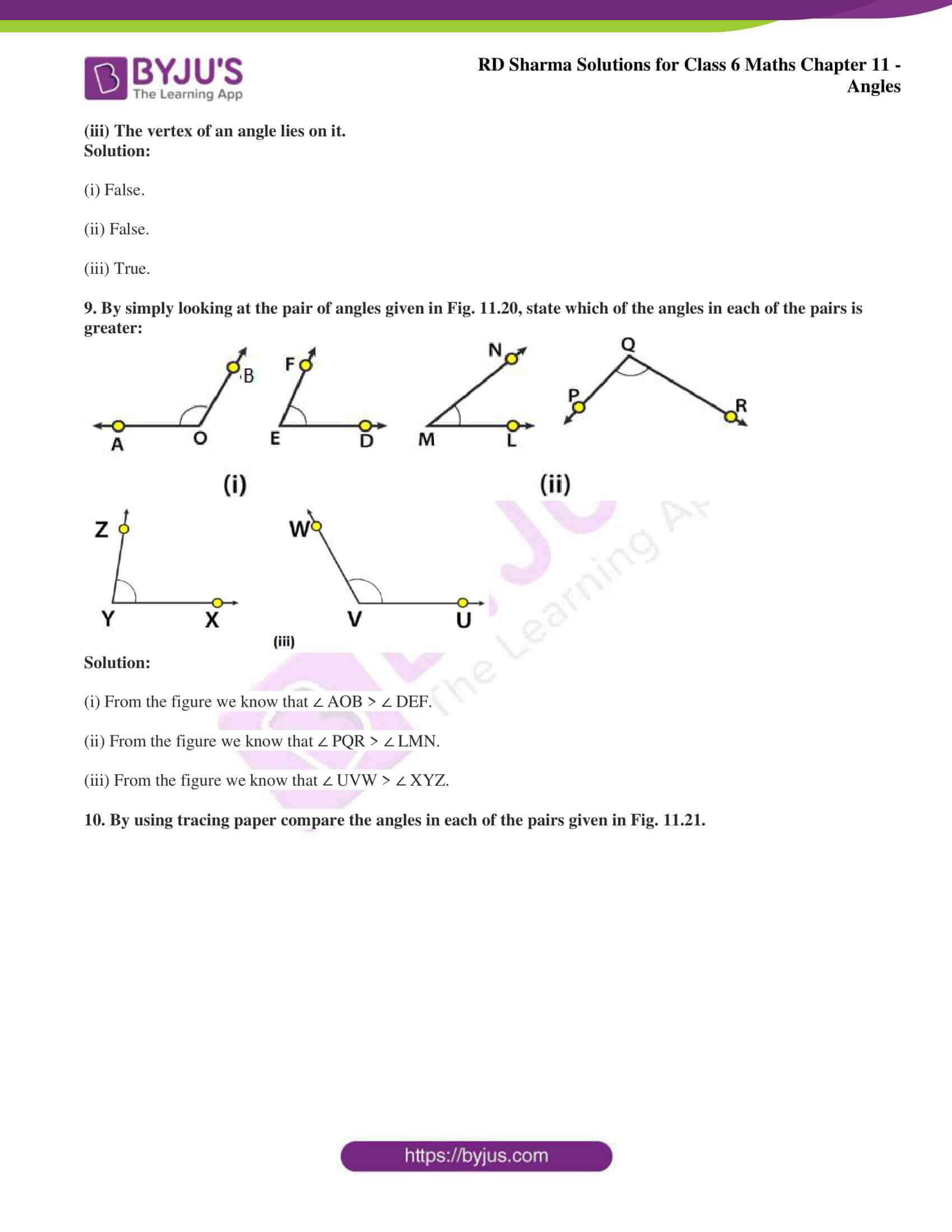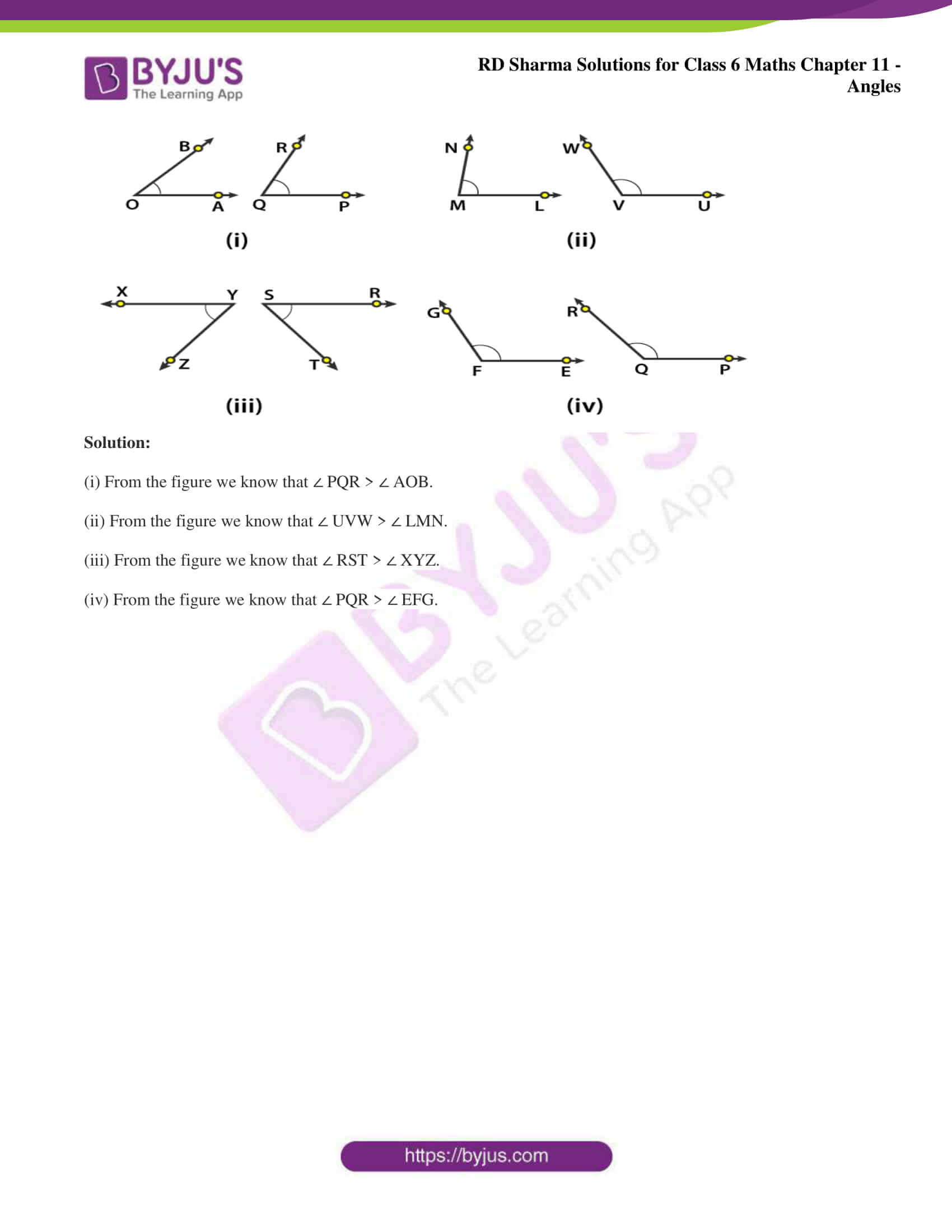### Access answers to Maths RD Sharma Solutions for Class 6 Chapter 11: Angles Exercise 11.1

1. Give three examples of angles from your environment.

Solution:

The three examples of angles are

The angle formed by the two adjacent fingers of our hand

The angle formed by two adjacent walls of a room

The angle formed by the hour hand and minute hand of a clock.

2. Write the arms and the vertex of ∠ LMP given in Fig. 11.14.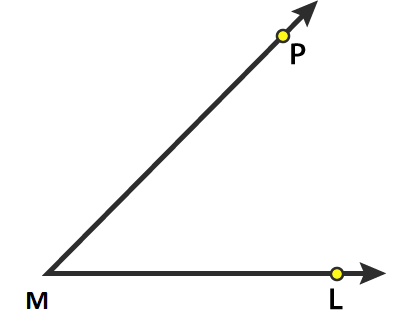Solution:

The vertex of ∠ LMP is M and the arms are ML and MP.

3. How many angles are formed in the figures 11.15 (i), (ii) and (iii)? Name them.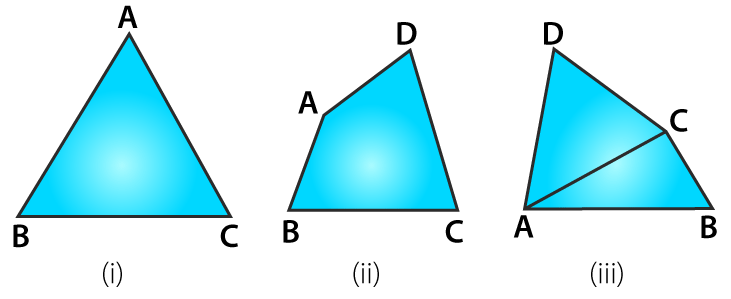Solution:

(i) Three angles are formed in figure (i) namely ∠ ABC, ∠ ACB and ∠ BAC.

(ii) Four angles are formed in figure (ii) namely ∠ ABC, ∠ BCD, ∠ CDA and ∠ DAB.

(iii) Eight angles are formed in figure (iii) namely ∠ ABC, ∠ BCD, ∠ CDA, ∠ DAB, ∠ CAB, ∠ CAD, ∠ BCA and ∠ ACD.

4. In Fig. 11.16, list the points which are: (i) in the interior of ∠ P (ii) in the exterior of ∠ P and (iii) lie on ∠ P.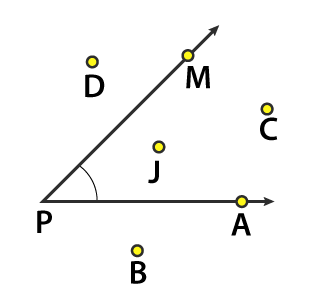Solution:

(i) The points which are in the interior of ∠ P are J and C.

(ii) The points which are in the exterior of ∠ P are B and D.

(iii) The points which lie on ∠ P are A, P and M.

5. In Fig. 11.17, write another name for: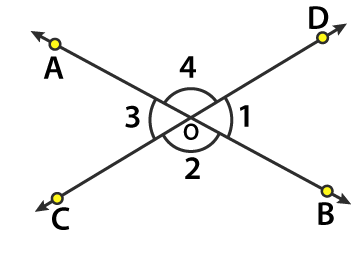(i) ∠ 1

(ii) ∠ 2

(iii) ∠ 3

(iv) ∠ 4

Solution:

(i) From the figure, another name for ∠ 1 is ∠ BOD or ∠ DOB.

(ii) From the figure, another name for ∠ 2 is ∠ BOC or ∠ COB.

(iii) From the figure, another name for ∠ 3 is ∠ COA or ∠ AOC.

(iv) From the figure, another name for ∠ 4 is ∠ AOD or ∠ DOA.

6. In Fig. 11.18, write another name for: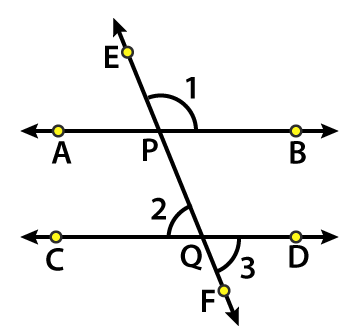(i) ∠ 1

(ii) ∠ 2

(iii) ∠ 3

Solution:

(i) From the figure, another name for ∠ 1 is ∠ EPB or ∠ BPE.

(ii) From the figure, another name for ∠ 2 is ∠ CQP or ∠ PQC.

(iii) From the figure, another name for ∠ 3 is ∠ DQF or ∠ FQD.

7. In Fig. 11.19, which of the following statements are true: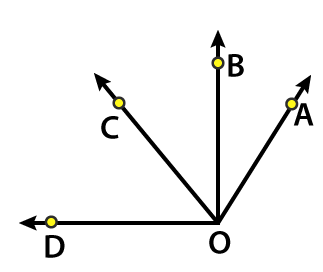(i) Point B is the interior of ∠ AOB.

(ii) Point B is the interior of ∠ AOC.

(iii) Point A is the interior of ∠ AOD.

(iv) Point C is the exterior of ∠ AOB.

(v) Point D is the exterior of ∠ AOC.

Solution:

(i) False. B lies on ∠ AOB.

(ii) True

(iii) False. A lies on ∠ AOD.

(iv) True

(v) True

8. Which of the following statements are true:

(i) The vertex of an angle lies in its interior.

(ii) The vertex of an angle lies in its exterior.

(iii) The vertex of an angle lies on it.

Solution:

(i) False.

(ii) False.

(iii) True.

9. By simply looking at the pair of angles given in Fig. 11.20, state which of the angles in each of the pairs is greater: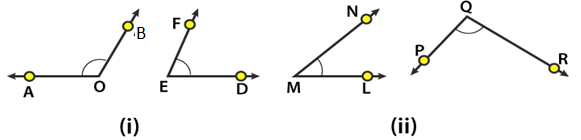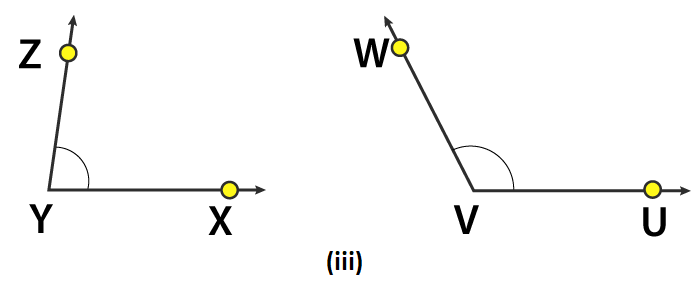Solution:

(i) From the figure we know that ∠ AOB > ∠ DEF.

(ii) From the figure we know that ∠ PQR > ∠ LMN.

(iii) From the figure we know that ∠ UVW > ∠ XYZ.

10. By using tracing paper compare the angles in each of the pairs given in Fig. 11.21.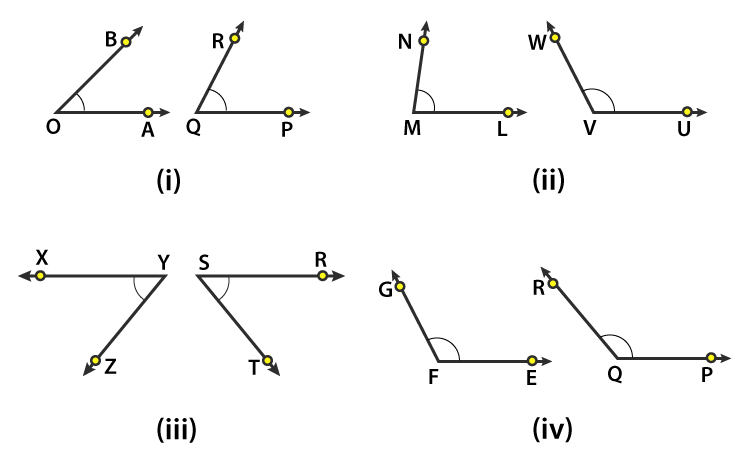Solution:

(i) From the figure we know that ∠ PQR > ∠ AOB.

(ii) From the figure we know that ∠ UVW > ∠ LMN.

(iii) From the figure we know that ∠ RST > ∠ XYZ.

(iv) From the figure we know that ∠ PQR > ∠ EFG.